###Show Posts

This section allows you to view all posts made by this member. Note that you can only see posts made in areas you currently have access to.

### Topics - Power

Pages:  2
1
##### Python / How Can i get Started with SFML On python (Is it a bad idea?)
« on: January 10, 2021, 04:29:35 pm »
Hello,

I am familiar with SFML, C++ CodeBlocks user,
I am starting a new project that require Python and i would like to use all the Graphical option SFML offer (within my Python),

How get i started? Do i have to add the libraires for SFML the way i did with CodeBlocks ? (https://www.sfml-dev.org/tutorials/2.5/start-cb.php) Or Do i have to do anything else?

Thanks

2
##### General / (solved) I am back after a long pause, i need to reconfigure CodeBlocks
« on: September 27, 2020, 08:41:35 pm »
Hello,
I lost one hard drive called "D:\" last month, it has all my codes, i could try to get my files back but as for now the hard drive is dead.
It has also my "project templates".

I just reconfigured the libraries ( https://www.sfml-dev.org/tutorials/2.5/start-cb.php) and tried to run the test code :
#include <SFML/Graphics.hpp>

int main()
{
sf::RenderWindow window(sf::VideoMode(200, 200), "SFML works!");
sf::CircleShape shape(100.f);
shape.setFillColor(sf::Color::Green);

while (window.isOpen())
{
sf::Event event;
while (window.pollEvent(event))
{
if (event.type == sf::Event::Closed)
window.close();
}

window.clear();
window.draw(shape);
window.display();
}

return 0;
}

I get this error message :
(image)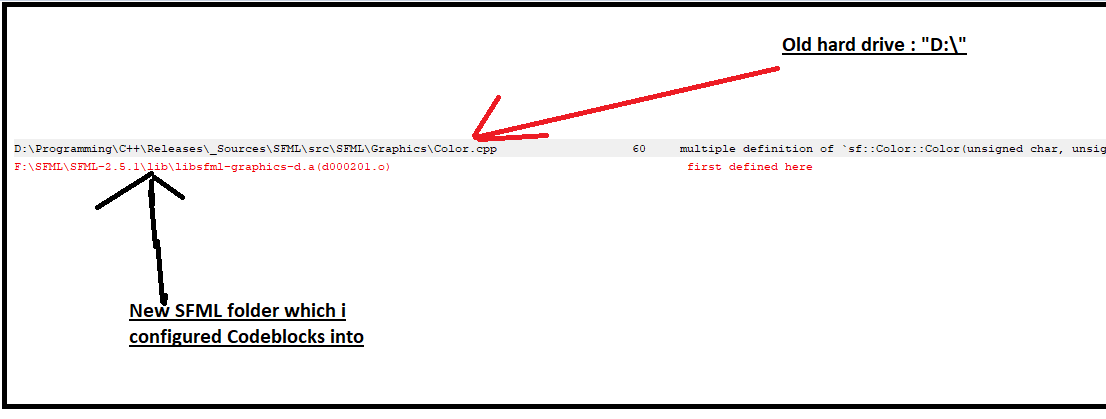Could someone help?
I tried to check on all codeblocks parameters and did not find anything related to "D:\", then i tried to check my windows variables (PATH stuff), same thing i did not see anything related SFML.
Thanks

3
##### Graphics / using push_back to modify only one parameter of the vertexAconstructor (setter?)
« on: June 01, 2020, 03:27:24 pm »
Hello,
I took a pause from SFML a bit, but i came back to do something briefly.
I wanted to modify colors of a vertexArray randomly.
1. VertexArray is already declared, the vertices positions are known.
2. The function takes the VertexArray as a parameter and modify the vertices colors. Its a "ref".

bool VertexColorREAL(sf::VertexArray &VV )
{

std::size_t cnt=VV.getVertexCount(); ///count
if(cnt==4)
{
sf::Color &c0=VV.color;
sf::Color &c1=VV.color;
sf::Color &c2=VV.color;
sf::Color &c3=VV.color;

c0.r = rand() % 255+ 0;
c0.g = rand() % 255+ 0;
c0.b = rand() % 255+ 0;
c1.r = rand() % 255+ 0;
c1.g = rand() % 255+ 0;
c1.b = rand() % 255+ 0;
c2.r = rand() % 255+ 0;
c2.g = rand() % 255+ 0;
c2.b = rand() % 255+ 0;
c3.r = rand() % 255+ 0;
c3.g = rand() % 255+ 0;
c3.b = rand() % 255+ 0;
}
if(cnt==3)
{
//..
}

return true;
}

3. The function checks the number of vertices (if count==4).. etc

Is it possible to work with push_back, to modify only the colors ? I am trying to write something like this :

bool VertexColorREAL(sf::VertexArray &VV )
{
vector<sf::Vertex> vertices;

for(int i=0;i<VV.getVertexCount();i++)
{
&vertices.push_back(sf::Vertex(sf::Color::Black)); // obviousely ERROR
/// I am not quite sure how to do it.
}

-> I am not quite sure how to do it, i don't know if push_back can be used as a setter somehow, the only use i know of is as a constructor, something like this :
vector<sf::Vertex> vertices; ///  (VECTOR  std c++)
vertices.push_back(sf::Vertex(sf::Vector2f(110.f,110.f),sf::Color::Black));
vertices.push_back(sf::Vertex(sf::Vector2f(210.f,110.f),sf::Color::Yellow));
vertices.push_back(sf::Vertex(sf::Vector2f(210.f,210.f),sf::Color::Blue));

Is there a way to turn around this as a color setter instead of constructors?
Thanks

4
##### Graphics / Inhereting from Transformable+Drawable+VertexArray: getBounds/draw error
« on: April 24, 2020, 05:51:34 pm »
Hello,
I don't know if this should be in the "window help" section or here, but the problem i am presensting here, started when i wanted to get the Bounding boxes of my entities.

I made a class using vertices and wanted to get the bounding boxes through the function "getBounds" by inhereting the class from the "VertexArray" class, like this :
class VertexClass :  public sf::VertexArray, public sf::Transformable , public sf::Drawable
{
private: /// ///////////////////////////////////////////
sf::VertexArray V;
sf::Texture Tex;
virtual void draw(sf::RenderTarget& target, sf::RenderStates states) const    {
states.transform *= getTransform();
states.texture = &Tex;
//states.texture = nullptr;
target.draw(V, states);
}
public:/// ////////////////////////////////////////////////////////////////////////////////////////////////
bool VertexFunction(sf::Vector2f v0,sf::Vector2f v1,sf::Vector2f v2 ) //(...,PrimitiveType type)
{
V.setPrimitiveType(sf::Triangles);
V.resize(3);

V.position = v0;
V.position = v1;
V.position = v2;
V.texCoords = v0;
V.texCoords = v1;
V.texCoords = v2;
return true;
}
};

One problem when calling the "draw" function :
//main
VertexClass VClass;
VClass.VertexFunction(sf::Vector2f(200,200),sf::Vector2f(400,200),sf::Vector2f(400,400));

....
window.draw(VClass);

Error message is :
Quote
'sf::Drawable' is an ambiguous base of 'VertexClass'

I searched on the internet and found these solutions : https://www.ibm.com/support/knowledgecenter/en/SSLTBW_2.1.0/com.ibm.zos.v2r1.cbclx01/cplr138.htm

Maybe i did not get it but i tried the following options :
window.sf::Drawable::draw(VClass);
//or
window.sf::VertexClass::draw(VClass);
I tried then to modify the class declaration, and added "sf::Drawable" before the draw function declaration. Still have the problem..

1) So how do we solve this situation if anyone knows?
2) Is there a better method to get the bound box of my verticesClass? (This is way i choose to put the post in the graphics section of the forum).

5
##### General / Free Textures/ images/ icons/ musics/ sounds /songs..
« on: April 20, 2020, 05:12:10 pm »
Hello,
Do you have a list of websites where you can get your hands on free Textures, or any kind of images (small for chars to big for backgrounds). Same thing for sounds or musics?

I mean, material you can you use freely in a game that would sell.. someday.

6
##### General discussions / Transforming your project into a real game (Android /Flash game / other..), ELI5
« on: April 20, 2020, 04:56:40 pm »
Hello,

I) Once your SFML C++ game is done, coded, compiled and ready to play by using the .exe file,

1) How far are you from being able to put it on the Google App Store?
A) I know you have to pay few bucks, like 100€/\$ a year?
B) I think you have to use something called android studio? Wrong?
C) You have to make sure you have no copyrighted material ? (music/images..)
D) ..

2) Making it into a flash game, is possible? Or do you have to had coded it in other langages?

A) Present it to some videogame website and ask them for a review? Or Youtuber/blog
B) Ask for SFML forum memebrs for support
C) Sell it for some company making games that would advertise it and get like a 50% cut revenues?

7
##### Graphics / (Solved) Splitting the screen with sf::View
« on: April 19, 2020, 03:51:03 pm »
Hello,
The non spiltted window shows as this :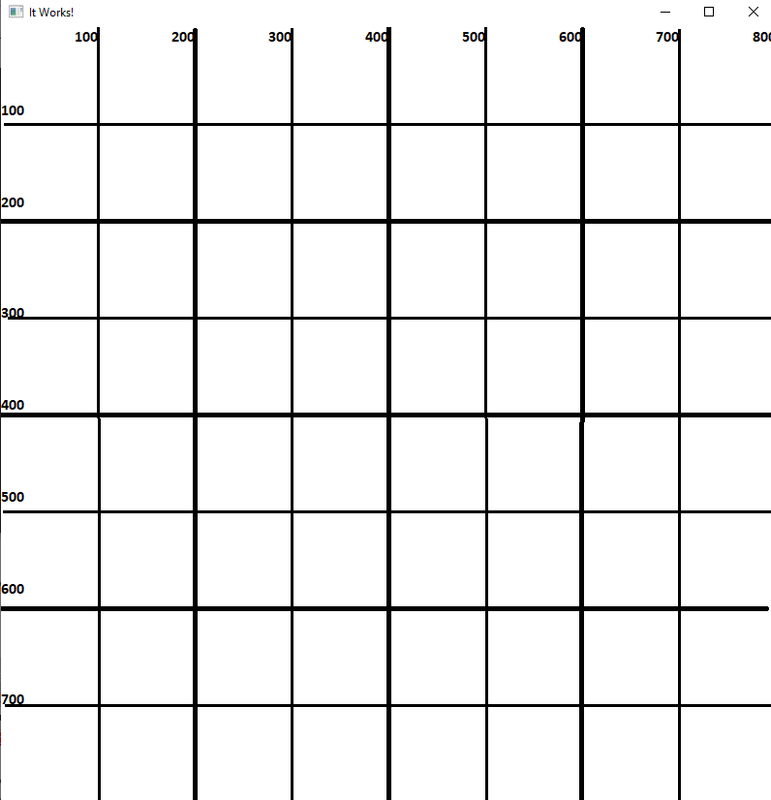The entity that is drawn is the grid, it is a rectangur shape called RS, you will see it mentioned at the end of post.

I try to split the screen in half by having 2 views in the same window like this  (view3b is the main view)
if(event.type == sf::Event::KeyPressed && event.key.code == sf::Keyboard::D) // Double view
{
sf::View view3bSave=view3b; // i save a copy of the main view)
//   player 1 (left side of the screen)
view3b.setViewport(sf::FloatRect(0.f, 0.f, 0.5f, 1.f));
window.setView(view3b);
// player 2 (right side of the screen)
view3bSave.setViewport(sf::FloatRect(0.5f, 0.f, 0.5f, 1.f));
window.setView(view3bSave);
}

And i get only the right part :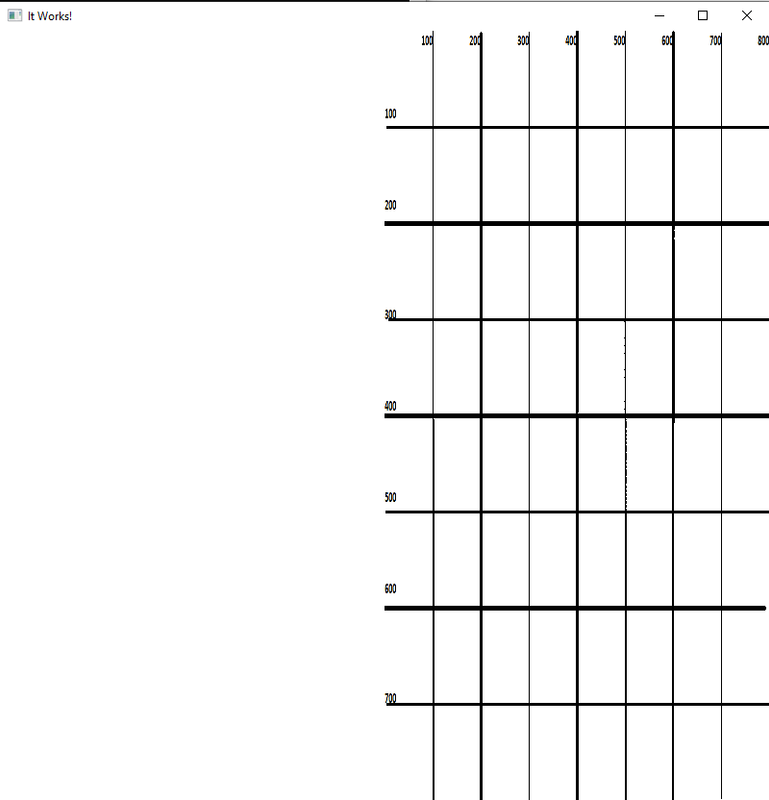Then i read this post : https://en.sfml-dev.org/forums/index.php?topic=11526.0
Where it is expalained the "window.draw(world);" has to be done following each "setView".
I tried to draw the rectangular shape (the grid) RD, as following.

if(event.type == sf::Event::KeyPressed && event.key.code == sf::Keyboard::D) // Double view
{
sf::View view3bSave=view3b;
//   player 1 (left side of the screen)
view3b.setViewport(sf::FloatRect(0.f, 0.f, 0.5f, 1.f));
window.setView(view3b);
window.draw(RS);
// player 2 (right side of the screen)
view3bSave.setViewport(sf::FloatRect(0.5f, 0.f, 0.5f, 1.f));
window.setView(view3bSave);
window.draw(RS);

}

It did not work ! By the way here is the declaration of the main View :
sf::View view3b;
view3b.reset(sf::FloatRect(0.f, 0.f, 800.f, 800.f));
// its the size of the window.
What am i missing?

8
##### Graphics / (Solved) Expanding size of rotated quads vertices - my method
« on: April 11, 2020, 03:14:54 am »
Hello,
I used a pretty long code to achieve what i wanted, meaning : being able to rotate, move AND expand an item made of vertices in any situation (whichever is value of the rotation angle).
I want first to explain the method i used, then i would like to ask hero members and non hero members, to show me how would they have achieved that with OTHER METHODS? Maybe some advanced methods/functions, or just different methods, i think discovering your methods for doing it might enlarge my understanding and offer a new view in programming, right now i just had to spend some with a paper and a pen to figure out how to achieve the expand item at any rotation angle, but i am pretty sure you guys know way faster methods to do it. So this is the purpose of this topic.
My method :

CenterObj.position=sf::Vector2f(300,300);
CenterObj.position=sf::Vector2f(400,300);
CenterObj.position=sf::Vector2f(400,400);
CenterObj.position=sf::Vector2f(300,400);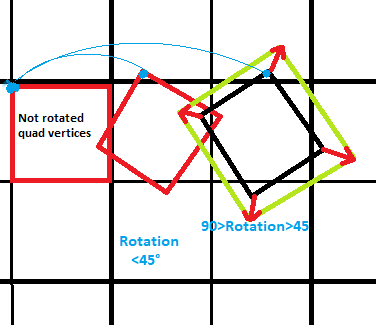Then i made a function that would "expand" it (increase the size of the quad vertices overall), it depends on the angle of rotation, the global rotation angle is saved in a global variables called (save) which grows any time the rotate function is called.
ang=0;
save=ang;
..
if (sf::Keyboard::isKeyPressed(sf::Keyboard::Q))
{
ang=4;
pivot(CenterObj,ang,4,CenterObj);
save=save+ang;
}
The rotation function (pivot) here is not important.

If the global rotation(save) is between 0 and 45, the expand function would behave in a certain manner, if the value of the global rotation (save) is between 45 and 90, it would behave in a different manner, same thing if it is between 90 and 135 and finally  between 135 and 180. if save is above 179 it will go back to 0 (using "%"). So we have many areas.. many "zones"
Last observation, all this regions are reversed if the "save" is between 180 and 360.

float zone=save%180;
So in each case i will calculate 2 variables i call "differenceX" and "differenceY", and these 2 variables are the ones that are used to change the values of vertices. This image show them, the algorithm is just below the picture :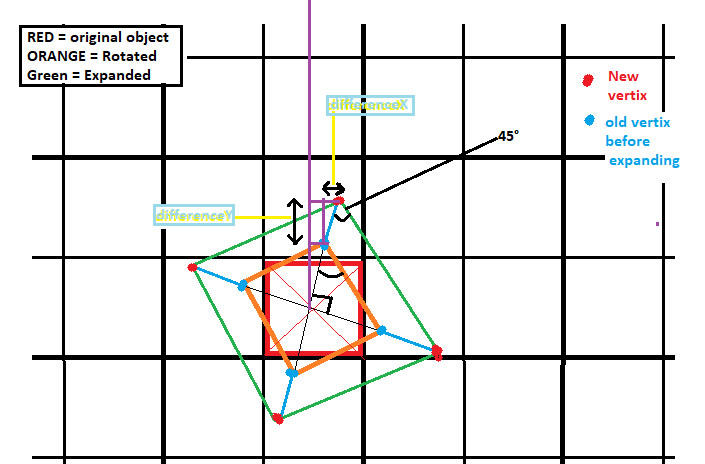void NewExpand(sf::VertexArray &V,float ExpandValue,char item,int NumberOfVertices)
{
//save=ang;
float zone=save%180;
//    cout << "save : " << save << endl;
cout << " zone(real angle) : " << zone << endl;
if (V.getVertexCount()==4)
{
if(zone==0)
{
V.position.x=V.position.x-ExpandValue; V.position.y=V.position.y-ExpandValue;
V.position.x=V.position.x+ExpandValue; V.position.y=V.position.y-ExpandValue;
V.position.x=V.position.x+ExpandValue; V.position.y=V.position.y+ExpandValue;
V.position.x=V.position.x-ExpandValue; V.position.y=V.position.y+ExpandValue;
float test = V.position.x;
//      cout << "value of of the quad vertices (case 0): " << test << " " << V.position.x << " " << V.position.x << " " << V.position.x << endl;
}
else if(zone>0&&zone<45){ Angle2=45-save; /// /zone A
float PivotedSideRectangle = sqrt((V.position.y-V.position.y)*(V.position.y-V.position.y) + (V.position.x-V.position.x)*(V.position.x-V.position.x)) ;
OldHypotenuse=COS(45)*PivotedSideRectangle;
float NewHypotenuse=OldHypotenuse+ExpandValue;
//        float ExpandedSideNewRect=NewHypotenuse/COS(45); //Idk if this is useful
float NewY=NewHypotenuse*COS(Angle2); // It's Y on the window eventhouth cosinus
float NewX=NewHypotenuse*SIN(Angle2);
float Ypart1=COS(Angle2)*OldHypotenuse;
float Xpart1=SIN(Angle2)*OldHypotenuse;
float differenceY=abs(NewY-Ypart1);
float differenceX=abs(NewX-Xpart1);
if(save%360>=180)
{
V.position.x=V.position.x+differenceX;
V.position.y=V.position.y+differenceY;
///
V.position.x=V.position.x-differenceY;
V.position.y=V.position.y+differenceX;
///
V.position.x=V.position.x-differenceX;
V.position.y=V.position.y-differenceY;
///
V.position.x=V.position.x+differenceY;
V.position.y=V.position.y-differenceX;
}
else{
V.position.x=V.position.x-differenceX;
V.position.y=V.position.y-differenceY;
///
V.position.x=V.position.x+differenceY;
V.position.y=V.position.y-differenceX;
///
V.position.x=V.position.x+differenceX;
V.position.y=V.position.y+differenceY;
///
V.position.x=V.position.x-differenceY;
V.position.y=V.position.y+differenceX;
}
//       cout << "value of of the quad vertices (case <45) : " << V.position.x << " " << V.position.x << " " << V.position.x << " " << V.position.x << endl;
}
else if(zone==45)
{
if(save%360>=180)
{
V.position.y=V.position.y+ExpandValue;
V.position.x=V.position.x-ExpandValue;
V.position.y=V.position.y-ExpandValue;
V.position.x=V.position.x+ExpandValue;
}
else
{
V.position.y=V.position.y-ExpandValue;
V.position.x=V.position.x+ExpandValue;
V.position.y=V.position.y+ExpandValue;
V.position.x=V.position.x-ExpandValue;
}}

else if(zone>45&&zone<90) /// Zone A2
{Angle2=save-45;
double PivotedSideRectangle = sqrt((V.position.y-V.position.y)*(V.position.y-V.position.y) + (V.position.x-V.position.x)*(V.position.x-V.position.x)) ;
OldHypotenuse=COS(45)*PivotedSideRectangle;
float NewHypotenuse=OldHypotenuse+ExpandValue;
float NewY=NewHypotenuse*COS(Angle2); // It's Y on the window eventhouth cosinus
float NewX=NewHypotenuse*SIN(Angle2);
float Ypart1=COS(Angle2)*OldHypotenuse;
float Xpart1=SIN(Angle2)*OldHypotenuse;
double differenceY=abs(NewY-Ypart1);
double differenceX=abs(NewX-Xpart1);
//            cout << PivotedSideRectangle << endl;
//            cout << OldHypotenuse << endl;
if(save%360>=180)
{
V.position.x=V.position.x-differenceX;
V.position.y=V.position.y+differenceY;
///
V.position.x=V.position.x-differenceY;
V.position.y=V.position.y-differenceX;
///
V.position.x=V.position.x+differenceX;
V.position.y=V.position.y-differenceY;
///
V.position.x=V.position.x+differenceY;
V.position.y=V.position.y+differenceX;
}
else
{
V.position.x=V.position.x+differenceX;
V.position.y=V.position.y-differenceY;
///
V.position.x=V.position.x+differenceY;
V.position.y=V.position.y+differenceX;
///
V.position.x=V.position.x-differenceX;
V.position.y=V.position.y+differenceY;
///
V.position.x=V.position.x-differenceY;
V.position.y=V.position.y-differenceX;
//       cout << "value of of the quad vertices (Case under 90) : " << V.position.x << " " << V.position.x << " " << V.position.x << " " << V.position.x << endl;
//       cout << "value of of the quad vertices.Y (Case under 90) : " << V.position.y << " " << V.position.y << " " << V.position.y << " " << V.position.y << endl;
}}
else if(zone==90)
{
if(save%360>=180)
{
V.position.x=V.position.x-ExpandValue;
V.position.y=V.position.y-ExpandValue;
V.position.x=V.position.x+ExpandValue;
V.position.y=V.position.y+ExpandValue;
}
else
{
V.position.x=V.position.x+ExpandValue;
V.position.y=V.position.y+ExpandValue;
V.position.x=V.position.x-ExpandValue;
V.position.y=V.position.y-ExpandValue;
}}
else if(zone>90&&zone<135)
{Angle2=90+45-save; /// //Zone B
double PivotedSideRectangle = sqrt((V.position.y-V.position.y)*(V.position.y-V.position.y) + (V.position.x-V.position.x)*(V.position.x-V.position.x)) ;
OldHypotenuse=COS(45)*PivotedSideRectangle;
float NewHypotenuse=OldHypotenuse+ExpandValue;
float ExpandedSideNewRect=NewHypotenuse/COS(45); //Idk if this is useful
float NewY=NewHypotenuse*COS(Angle2); // It's Y on the window eventhouth cosinus
float NewX=NewHypotenuse*SIN(Angle2);
float Ypart1=COS(Angle2)*OldHypotenuse;
float Xpart1=SIN(Angle2)*OldHypotenuse;
float differenceY=abs(NewY-Ypart1);
float differenceX=abs(NewX-Xpart1);
//        cout << "angle2 :" << Angle2 << endl;
if(save%360>=180)
{
V.position.x=V.position.x+differenceY;
V.position.y=V.position.y-differenceX;
V.position.x=V.position.x+differenceX;
V.position.y=V.position.y+differenceY;
V.position.x=V.position.x-differenceY;
V.position.y=V.position.y+differenceX;
V.position.x=V.position.x-differenceX;
V.position.y=V.position.y-differenceY;
}
else
{
V.position.x=V.position.x-differenceY;
V.position.y=V.position.y+differenceX;
V.position.x=V.position.x-differenceX;
V.position.y=V.position.y-differenceY;
V.position.x=V.position.x+differenceY;
V.position.y=V.position.y-differenceX;
V.position.x=V.position.x+differenceX;
V.position.y=V.position.y+differenceY;
//          cout << "value of of the quad vertices (Case under 135) : " << V.position.x << " " << V.position.x << " " << V.position.x << " " << V.position.x << endl;
//          cout << "value of of the quad vertices.Y (Case under 135) : " << V.position.y << " " << V.position.y << " " << V.position.y << " " << V.position.y << endl;

}}
else if(zone==135)
{
if(save%360>=180)
{
V.position.y=V.position.y-ExpandValue;
V.position.x=V.position.x+ExpandValue;
V.position.y=V.position.y+ExpandValue;
V.position.x=V.position.x-ExpandValue;
}
else
{
V.position.y=V.position.y+ExpandValue;
V.position.x=V.position.x-ExpandValue;
V.position.y=V.position.y-ExpandValue;
V.position.x=V.position.x+ExpandValue;
}}
else if(zone>135&&zone<180){Angle2=save-(45+90);
float PivotedSideRectangle = sqrt((V.position.y-V.position.y)*(V.position.y-V.position.y) + (V.position.x-V.position.x)*(V.position.x-V.position.x)) ;
OldHypotenuse=COS(45)*PivotedSideRectangle;
float NewHypotenuse=OldHypotenuse+ExpandValue;
float NewY=NewHypotenuse*COS(Angle2); // It's Y on the window eventhouth cosinus
float NewX=NewHypotenuse*SIN(Angle2);
float Ypart1=COS(Angle2)*OldHypotenuse;
float Xpart1=SIN(Angle2)*OldHypotenuse;
float differenceY=abs(NewY-Ypart1);
float differenceX=abs(NewX-Xpart1);

if(save%360>=180)
{
V.position.x=V.position.x+differenceY;
V.position.y=V.position.y+differenceX;
V.position.x=V.position.x-differenceX;
V.position.y=V.position.y+differenceY;
V.position.x=V.position.x-differenceY;
V.position.y=V.position.y-differenceX;
V.position.x=V.position.x+differenceX;
V.position.y=V.position.y-differenceY;}
else {
V.position.x=V.position.x-differenceY;
V.position.y=V.position.y-differenceX;
V.position.x=V.position.x+differenceX;
V.position.y=V.position.y-differenceY;
V.position.x=V.position.x+differenceY;
V.position.y=V.position.y+differenceX;
V.position.x=V.position.x-differenceX;
V.position.y=V.position.y+differenceY;
//        cout << "value of of the quad vertices (Case under 180) : " << V.position.x << " " << V.position.x << " " << V.position.x << " " << V.position.x << endl;
//        cout << "value of of the quad vertices.Y (Case under 180) : " << V.position.y << " " << V.position.y << " " << V.position.y << " " << V.position.y << endl;
}
}
} /// end of : if (V.getVertexCount()==4)
} /// end of function

Inside the main, i will call the rotate function "pivot" and check the value of angle, so at each zone i will test the 'NewExpand" function and see if it works. (as shown in the second code of this post, using the letter "Q", check above). Well it does!I am proud to have achieved it. Now i would be happy to see how you do it guys? I have no doubt there are much better and faster and more powerful methods to associate an expand function with a rotate and a movement one for a groupe of vertices or other, with a result expeceted similar to this one :

https://gfycat.com/poisedthinhartebeest

9
##### Graphics / [SOLVED for now]Why reducing a vertices object size is reversed at some point ?
« on: April 09, 2020, 06:56:29 pm »
Hello again,
I made a function to reduce or contract the size of a shape made of vertices :
void contract(sf::VertexArray &V,float ExpandValue,char item,int NumberOfVertices)

It seems to be working, but i noticed the shape start to expanding after it has reached a size of 0 , like this :
https://gfycat.com/fr/illfatedesteemedenglishpointer

The window is a (800,800).

Thanks
P~

10
##### System / (SOLVED)window.pollEvent=>Only 1 action can be treated at once?(Move AND Rotate)
« on: April 09, 2020, 03:29:28 pm »
Hello,
I made a program where you can press the arrow keys to move an item, and if you press "Q" the item would rotate,

I noticed i could not have the item MOVE and rotate at the same time if i pressed an arrow key and 'Q' at the same time. Is that normal?

Here is the code :

while (window.isOpen())
{

...
...
sf::Event event;
while (window.pollEvent(event))
{
if(event.type==sf::Event::KeyPressed&&event.key.code==sf::Keyboard::Q)
{
pivot(RightObj,ang,sf::Vector2f(saveCenter1,saveCenter2),3);
pivot(LeftObj,ang,sf::Vector2f(saveCenter1,saveCenter2),3);

// save= ang+save;

}
if(event.type==sf::Event::KeyPressed&&event.key.code==sf::Keyboard::Right)
{

shiftVertex(RightObj,10,'r',3);
shiftVertex(LeftObj,10,'r',3);

}

...
...
}

It's quiiite frustrating, i want to make a reeally dynamic game where you can press many keys and ave many actions happen.

11
##### General / Hide the files of your "game" or "app"
« on: April 09, 2020, 02:33:26 pm »
Hello,
I am very excited for learning SFML, and still have many questions,
On a video tutorial, i saw how a compiled programme through "release" option, could be generated and be distributed, the tutorial mentionned not to forget any data files (font/image/texture/musics).

And i tried it, i could send a programme.exe + the files + the .dll files all in one package, the receiver extracted all and was able to test my programme.

Now is there a way to hide all the files/data from the user of the programme?

12
##### Java / Android studio - java - sfml
« on: April 09, 2020, 02:28:39 pm »
Hello,
I read JSFML developpent has stoped, i plan to learn java when i have time, would it still be possible then to use sfml on JAVA and android studio to make little smartphone app games?

Thanks,
Good day

13
##### Graphics / Using ::transform to rotate vertices
« on: April 09, 2020, 01:30:55 pm »
Hello,
My first big challenge as a newcomer was to make a concave shape rotate, thanks to A_Sentient_Tomato for the right matrix he shared (https://en.sfml-dev.org/forums/index.php?topic=26984.0), and especially to Hapax for providing this link : https://www.geeksforgeeks.org/2d-transformation-rotation-objects/

https://gfycat.com/fr/realisticwarpedamurstarfish

I used this matrix :
[cos(A)     -sin(A)]
[sin(A)      cos(A)]
Okay, and i know how we got it and what it means
I did not use any transform class , i used only codes of this form :
Quote from: A_Sentient_Tomato
let's say your shape's vertices are {(x,y), (x1, y1), (x2,y2)}
create a new shape with vertices at {(x-C, y-C), (x1-C, y1-C), (x2-C, y2-C)}
_______________________________________________________________
Topic :
Now my problem is this "transform" class thing and its functions https://www.sfml-dev.org/documentation/2.5.1/classsf_1_1Transform.php

____________________________________________________________
It has a 3x3 matrix i saw, here are my questions :

1) How is a 3x3 matrix relevant when we are only working on 2D spaces (sf::window has x and y coordinates only)?

2) is it possible to use the matrix 2x2 that i mentionned (with cos and sin, just above) with the "transform" class?

3) If you have anything to add, or examples about this transform class that is different from what is said on the tutorial, i'd like to hear please.

(My next challenge is to make vertices shapes "expand" in size.....)

Thanks for any enlightenment.

14
##### Audio / Can't play 2 "musics" on one game?
« on: April 06, 2020, 12:32:08 pm »
Hello,
While experimenting and making a little game, i wanted to start the game with a first music and further it at some point with a second one.

The musics work fine if the function music.play() is called before the while(window.isOpen()), but if i put a music.play() inside this "while" the music will not..play correctly. It will stop at every move the mouse cursor make. Meaning if the mouse hasn't moved the music plays, if the cursor moves, it will restart.

I decided then, to put a bool variable inside the  "while", and call the function music.play() after the while (after : while(window.isOpen() ) ). The condition would be for the bool variable to be true.

It does not work!

bool part2=0;
bool cond1=0;
bool part3=0;
...
..

///MUSIC
sf::Music Music2;
if(Music2.openFromFile("data/music2.ogg")==0)
{
return 1;
}

Music2.setVolume(30);
Music2.setLoop(true); ///
Music2.play();
//second music
sf::Music Music3;
if(Music3.openFromFile("data/music3.ogg")==0)
{
return 1;
}
Music3.setVolume(30);
Music3.setLoop(true); ///
Music3.play();
Music3.pause(); /// i tried here to make the music start before the while then pause it, to see if could just resume the music later.

while (window.isOpen())
{

sf::Event event;
while (window.pollEvent(event))
{
if (event.type == sf::Event::Closed)
window.close();

if (event.type== sf::Event::MouseButtonPressed && event.mouseButton.button ==sf::Mouse::Left)
{
....
...
{if(position.y<=446&&position.y>=263){if(money>=300&&weight<=450){part2=0;cond1=1;money=money-300;}}}

.....

Music3.play(); /// would do the problem discribed above

}

///LOGIC
....
...
//  if(weight<=450&&cond1==0){part2=1;}

if(cond1==1){part3=1; part2=0;}//Music2.stop();Music2.play();}  ... // it did not work here either
// if(Music2.getStatus()==sf::Sound::Stopped)
//{Music3.play();}  // does not play
...
..

15
##### Graphics / A total of 7 questions
« on: April 01, 2020, 12:10:39 am »
Hello,
I don't want to make a post for every question, maybe i should?

Here are all the questions i encountered so far, if someone could enlighten.. :

1) i reread almost all graphics tutorials except the "view" one, and i did not find a single example of a use of the a Transformable class with a matrix, i saw this on the tutorial page (https://www.sfml-dev.org/tutorials/2.5/graphics-transform.php)
sf::Transform t3(2.f, 0.f, 20.f,
0.f, 1.f, 50.f,
0.f, 0.f, 1.f);
I do understand how a Transform can "absorb" many transformation (Translate, rotate,scale...etc), and then merge the transform in a state or directly on a draw function.
But i have no clue what this matrix means?
I have been advised on another topic to use this matrix to do a rotation :
[cos(A)     -sin(A)]
[sin(A)      cos(A)]

I thought i could understand it later, but after rereading and trying to understand some SFML examples such the "tilemap" i still dont know what matrix have to do with Transformable.

2) ROTATION:
After re reading almost all turorials (except the "View" one) and trying to remember all graphics functions SFML have, i can finally say i dont know well how getRotation works.
Here an example of a rectangular shape with 2 other shapes i want to make them rotate AROUND the rectangle, the three shapes are named R (Rectangle), C(A triangle made with vertices) and O(and Object with a traingular shape, its the smallest and has one color, compared to the C triangle which is multi coloured).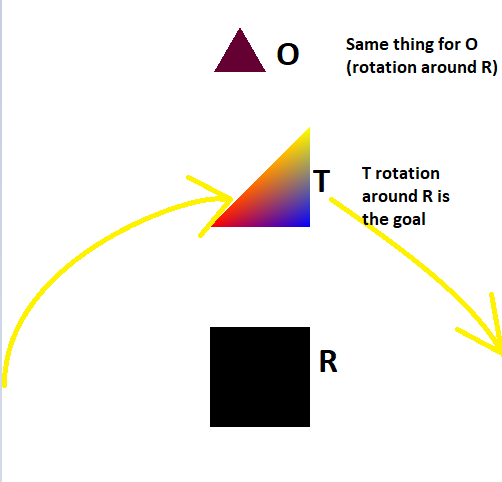sf::RenderWindow window(sf::VideoMode(800, 600), "It Works!", sf::Style::Close, contextSettings);
window.setFramerateLimit(30);

///SHAPE1
sf::RectangleShape R; // rectangle
R.setPosition(450,350);
R.setSize(sf::Vector2f(100,100));
R.setFillColor(sf::Color::Black);
///SHAPE2
sf::VertexArray V(sf::Triangles,3); //Triangle closest to the rectangle
V.position = sf::Vector2f(R.getPosition().x,R.getPosition().y-100); // which is (450,350)
V.position = sf::Vector2f(R.getPosition().x+100,R.getPosition().y-100); // which is (550,350)
V.position = sf::Vector2f(R.getPosition().x+100,R.getPosition().y-200); // which is (550,250)
V.color = sf::Color::Red;
V.color = sf::Color::Blue;
V.color = sf::Color::Yellow;
///SHAPE3
sf::CircleShape O(30,3); // farest shape : object

O.setPosition(R.getPosition().x,R.getPosition().y-300);
O.setFillColor(sf::Color(100,0,50));

//        newOctagon.setOutlineThickness(6.f);
//    newOctagon.setOutlineColor(sf::Color::Red);
O.setOrigin(R.getOrigin());
O.rotate(45);
//R.rotate(45);

// triangle.color = sf::Color::Red;

while (window.isOpen())
{

sf::Event event;
while (window.pollEvent(event))
{
if (event.type == sf::Event::Closed)
window.close();
}

window.clear(sf::Color::White);

window.draw(R);
window.draw(V);
window.draw(O);

window.display();

So is it possible to make O which is a CircleShape, rotate around R?
Is it possible to make T which is a vertex type, rotate around R?

3)
Is it possible to fill multiple colours for regular shapes(not vertices) choosing areas with "Rect" ? (
Example like this :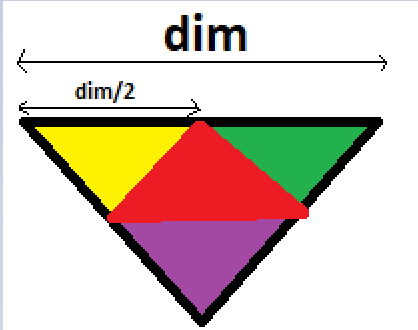4) On the class sf::Unit8
I am not sure How it it used, because i find no classe called "Unit8" browsing the documentation: here an example i saw somewhere :
sf::Uint8* pixels = new sf::Uint8[width * height * 1]; // * 4 because pixels have 4 components (RGBA)
//sf::Uint8* pixels = new sf::Uint8[width /2];
// sf::Uint8* pixels2 = sf::Vector2f(400,0)...; // get a fresh chunk of pixels (the next frame of a movie, for example)
tex1.update(pixels); /// FIRST PROB

What happens to the Texture tex1 here?

5) A random vertices generator does nor work inside a new "MyEntity" class while drawing it on the window, but the code works outside the new class (inside main), this:
int N;
cout << endl << " choose N : (below 26000) " << endl;
cin >> N;
sf::VertexArray Vexy(sf::TriangleStrip,N);
srand(time(nullptr)); // use current time as seed for random generator
for(int i;i!=N;i++)
{

int random_variable2 = rand()%400+1;
int random_variable3 = rand()%500+300;
Vexy[i].position = sf::Vector2f(random_variable2+random_variable3, random_variable2/2);
Vexy[i].color = colorArray[rand()%5];

}

Result :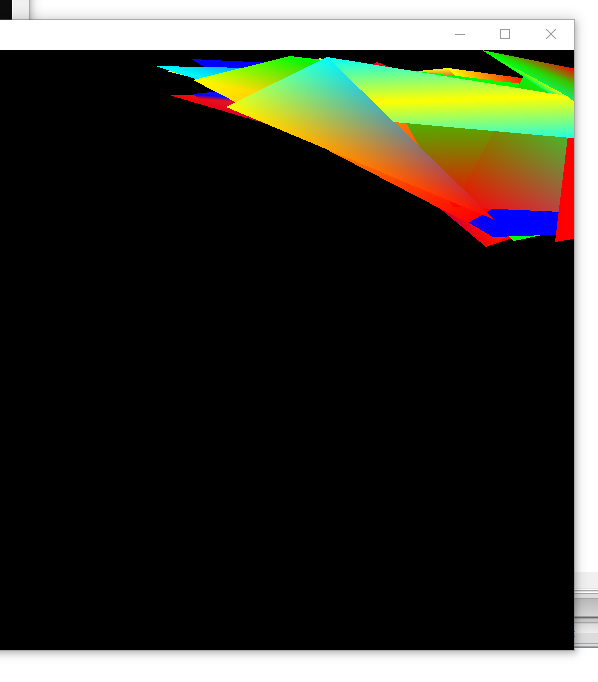But while i m using a new classe, like this one, it does not work(does not show up with the function draw)
using namespace std;
class MyEntity : public sf::Drawable, public sf::Transformable
{
public:

MyEntity(float NewPointcount) : PC(NewPointcount)
{
sf::VertexArray Vex(sf::TriangleStrip, PC);
const sf::Color colorArray={sf::Color::Cyan, sf::Color::Blue, sf::Color::Green, sf::Color::Red, sf::Color::Yellow };

///USING THE FUNCTION RAND => HASARD
srand(time(nullptr)); // use current time as seed for random generator
Vex.position = sf::Vector2f(0, 0);
Vex.color = colorArray[rand()%5];
for (int n=1; n != PC-1; ++n)
{
int random_variable = rand()%200+1;
int random_variable3 = rand()%600+300;
cout << "Random value on [0 " << RAND_MAX << "]: "
<< random_variable << '\n';

Vex[n].position = sf::Vector2f(random_variable, random_variable3);
cout << rand()%5 << endl;

Vex[n].color = colorArray[rand()%5];
}
Vex[PC-1].position =  sf::Vector2f(0, 0);
Vex[PC-1].color = colorArray[rand()%5];

};

private:

virtual void draw(sf::RenderTarget& target, sf::RenderStates states) const
{
// apply the entity's transform -- combine it with the one that was passed by the caller
states.transform *= getTransform(); // getTransform() is defined by sf::Transformable

// apply the texture
states.texture = &m_texture;

// you may also override states.shader or states.blendMode if you want

// draw the vertex array
target.draw(m_vertices, states);
}

sf::VertexArray m_vertices;
sf::Texture m_texture;
int PC;
};

and inside the main :
MyEntity ME(6);
..
window.clear(sf::Color::Black);
window.draw(ME);
window.display();

Nothings shows up. Also the difference between using the CLASS and the exemple before is that i set the first and last vertices outside the "for".

6) My last question is about the "Tilemap" example :
Which can be found at the bottom of this page : https://www.sfml-dev.org/tutorials/2.5/graphics-vertex-array.php

I had a hard time  undesrtanding the the variables tu and tv, i think a sentence should be written as comment to describe them, it's a google search on them and made me fall on one of the old posts from SFML forum and made me understand that : "tu and tv are the tile coordinates of the texture. tu is the column of the tile, and tv its line."

Okay what does this line exactly do?
m_vertices.resize(width * height * 4);
I can read here : https://www.sfml-dev.org/documentation/2.5.1/classsf_1_1VertexArray.php
that the resize function purpose is to change the number of "points" or "vertices".
Dont we always need 4-5 vertices to draw a tile? Which is rectangular?
I really dont get this line i keep finding everywhere : "(width * height * 4)"

7) Also, i did an experiment, with adding a "cout" to know the location of the FIRST point of each tile + the location of the first TexCoord for each said point :
quad.position = sf::Vector2f(i * tileSize.x, j * tileSize.y);
cout<< " position 1           :" << i * tileSize.x << "-" << j * tileSize.y << endl;
quad.position = sf::Vector2f((i + 1) * tileSize.x, j * tileSize.y);
quad.position = sf::Vector2f((i + 1) * tileSize.x, (j + 1) * tileSize.y);
quad.position = sf::Vector2f(i * tileSize.x, (j + 1) * tileSize.y);

// define its 4 texture coordinates
quad.texCoords = sf::Vector2f(tu * tileSize.x, tv * tileSize.y);
cout<< " coordinates TEX 1 :" << tu * tileSize.x<< "-" << tv * tileSize.y << endl;
quad.texCoords = sf::Vector2f((tu + 1) * tileSize.x, tv * tileSize.y);
quad.texCoords = sf::Vector2f((tu + 1) * tileSize.x, (tv + 1) * tileSize.y);
quad.texCoords = sf::Vector2f(tu * tileSize.x, (tv + 1) * tileSize.y);

And i noticed this :I still find it hard to understand it, i guess i will have to do many more examples to get it quicker, it seems that quad.position thing browses line by line after finishing a line one after another (from left to right), while quad.texCoors seems to browse vertically (from up to down then it changes the collumn)
Anyway this last question was not really a question.

Pages:  2